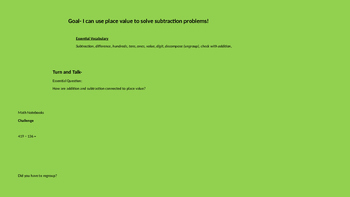# Subtracting three digit numbers using a variety of strategies PowerpointSubject
Resource Type
File Type
Presentation (Powerpoint) File (430 KB|23 pages)
Standards
\$5.00
• Product Description
• Standards
Subtracting three digit numbers using a variety of strategies

Place Value Blocks
Expanded Form
Number Line

-Whole group or small group
-Games
-Assessments
-Vocabulary talk
-Partner talk
Explain why addition and subtraction strategies work, using place value and the properties of operations.
Add and subtract within 1000, using concrete models or drawings and strategies based on place value, properties of operations, and/or the relationship between addition and subtraction; relate the strategy to a written method. Understand that in adding or subtracting three-digit numbers, one adds or subtracts hundreds and hundreds, tens and tens, ones and ones; and sometimes it is necessary to compose or decompose tens or hundreds.
Total Pages
23 pages